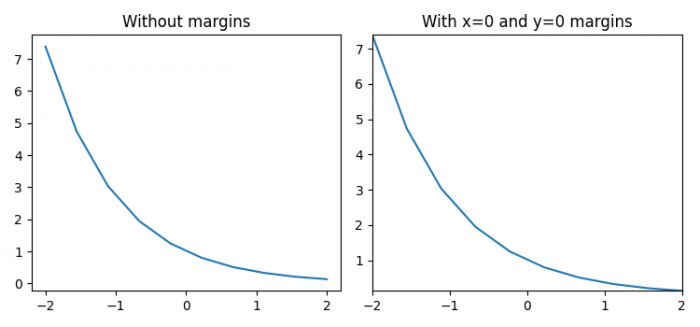# How to set the margins of a Matplotlib figure?

To set the margins of a matplotlib figure, we can use margins() method.

## Steps

• Set the figure size and adjust the padding between and around the subplots.
• Create t and y data points using numpy.
• Add a subplot to the current figure at index 1.
• Plot t and y data points using plot() method.
• Set the title of the plot.
• Add a subplot to the current figure at index 2.
• Plot t and y data points using plot() method.
• Set the title of the plot.
• Set margins of the plot using margins(x=0, y=0).
• To display the figure, use show() method.

## Example

import numpy as np
from matplotlib import pyplot as plt

plt.rcParams["figure.figsize"] = [7.50, 3.50]
plt.rcParams["figure.autolayout"] = True

t = np.linspace(-2, 2, 10)
y = np.exp(-t)
plt.subplot(121)
plt.plot(t, y)
plt.title("Without margins")
plt.subplot(122)
plt.plot(t, y)
plt.title("With x=0 and y=0 margins")
plt.margins(x=0, y=0)
plt.show()

## Output